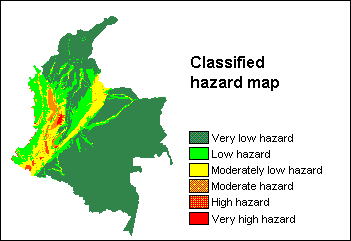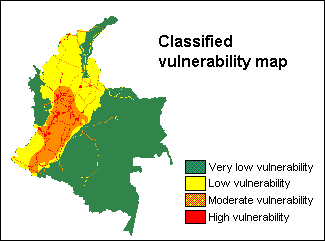# Summary

1. Hazard, vulnerability and risk analysis

This case study is intended to illustrate the meaning of hazard, vulnerability and risk, using a very simple data set on the national-scale of Colombia (South America). The occurrence of a disaster depends on two factors:

• hazard: the probability of occurrence of a potentially damaging phenomenon,
• vulnerability: the degree of loss resulting from the occurrence of the phenomenon.

You will first generate a qualitative hazard map by combining several factor maps. Then a vulnerability map is made. Finally, the hazard and the vulnerability map are combined into a risk map.

Creation of the hazard mapFirst, attribute tables are created for the following input maps: seismic hazards, landslide hazards, volcanic hazards, tsunami hazard, beach erosion/accumulation hazard. To all classes in these maps, different weight values are assigned in their attribute table.

Then, attribute maps of the weight values are derived from all these maps; these are the first factor maps.

Further, with mapcalc, factor maps are obtained from maps on inundation hazard, rivers, and for altitudes above 1000m.

Finally, all factor maps are summed with a mapcalc statement and a hazard map is obtained. The hazard map is then classified.

Creation of the vulnerability mapFirst, the population density of each department (province) in Colombia is calculated in a table: for each department the population figure has to be entered while the area of the departments is joined from the histogram of the departmental map. Population density is then calculated through a simple tabcalc formula.

Next, attribute tables are created for input maps depicting industrial regions, concentration of economic activities and main infrastructure. To all classes in these maps, weight values are entered in their attribute table. Subsequently attribute maps of the weight values are derived. These are the first factor maps.

In the Colombia table with the population densities, weight values are assigned through a tabcalc formula; then an attribute map is derived. This is another factor map. All factor maps are summed with a mapcalc formula and the vulnerability map is obtained. This vulnerability map is then classified.

Creation of the risk mapA two-dimensional table is created in which for each combination of hazard classes and vulnerability classes an output risk class is assigned.

The two-dimensional table is applied on the classified hazard map and the classified vulnerability map, and the risk map is obtained.

Quantification of risks per department

The Colombia map (with the Colombian departments) is crossed with the Risk map; in the cross table the number of pixels with high, medium and low risk can be seen for each Colombian department.

These number of pixels with high risk, medium risk and low risk are joined from the cross table into three separate columns of table Colombia. Further, the the total number of pixels per department is joined from the cross table into table Colombia by using a join with with an aggregation.

Finally, for each province, the percentage of high risk, medium risk and low risk is calculated with some tabcalc formulas.

For information on this case study, contact:

C.J. van Westen

Department of Earth Systems Analysis,

International Institute for Geo-Information Science and Earth Observation (ITC),

P.O. Box 6, 7500 AA Enschede, The Netherlands.

Tel: +31 53 4 874 263, Fax: +31 53 4 874 336, e-mail: westen@itc.nl